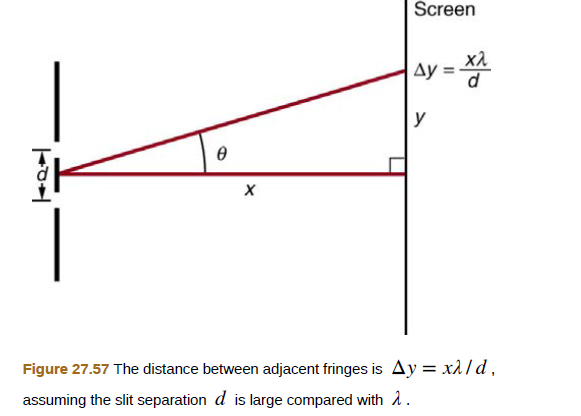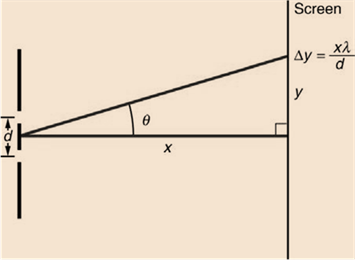Chapter 27, Problem 39PE

Chapter
Section
Textbook Problem

The analysis shown in the figure below also applies to diffraction gratings with lines separated by a distance d. What is the distance between fringes produced by a diffraction grating having 125 lines per centimeter for 600-nm light, if the screen is 1.50 m away?To determine

What is the distance between fringes produced by a diffraction grating having 125 lines per centimeter for 600-nm light, if the screen is 1.50 m away; the analysis shown in the figure below also applies to diffraction gratings with lines separated by a distance d.?Explanation

Given info:

λ=600nmN=125 lines/cmx=1.50m

Formula used:

Distance between fringes,

Δy=xλd

Calculation:

Let us find slit separation,

N=125lines/cmd=1

Still sussing out bartleby?

Check out a sample textbook solution.

See a sample solution

The Solution to Your Study Problems

Bartleby provides explanations to thousands of textbook problems written by our experts, many with advanced degrees!

Get Started

Find more solutions based on key concepts